# Nested VLOOKUP – Excel & Google Sheets

This tutorial will demonstrate how to perform a Nested VLOOKUP in Excel and Google Sheets. If you have access to the XLOOKUP Function, we recommend performing a nested XLOOKUP instead.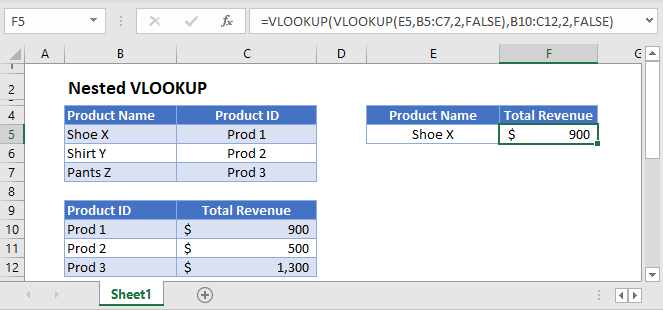If you need to perform a lookup that is dependent on looking up another value, you can use a Nested VLOOKUP formula:

``=VLOOKUP(VLOOKUP(E3,B3:C5,2,FALSE),B8:C10,2,FALSE)``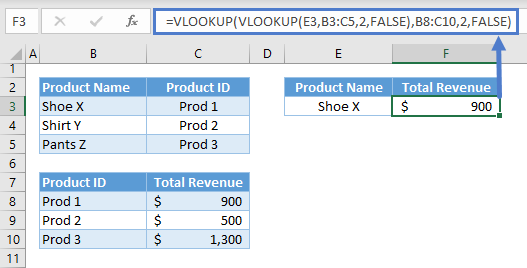Let’s walkthrough the formula:

First, we need to perform the 1st VLOOKUP to extract the required lookup value (e.g., Product ID) for our main VLOOKUP:

``=VLOOKUP(E3,B3:C5,2,FALSE)``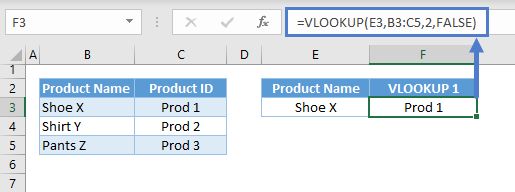Note: The VLOOKUP Function performs a vertical lookup in the first column of the table array starting from the top of the list going down (i.e., top-down). If it finds a match, it returns the corresponding value from the column described by the column index, and if not, it returns an error.

Next, we substitute the result to our main VLOOKUP and perform a lookup in another table:

``=VLOOKUP(F3,B8:C10,2,FALSE)``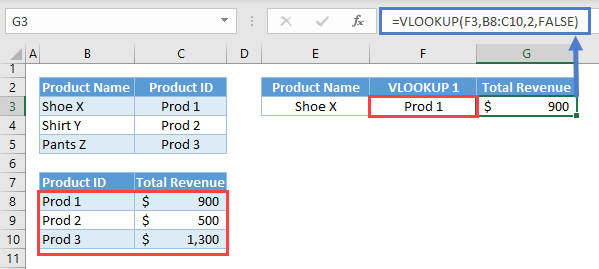Combining all of these results to our original formula:

``=VLOOKUP(VLOOKUP(E3,B3:C5,2,FALSE),B8:C10,2,FALSE)``

## Nested VLOOKUP in Google Sheets

The formula works the same way in Google Sheets: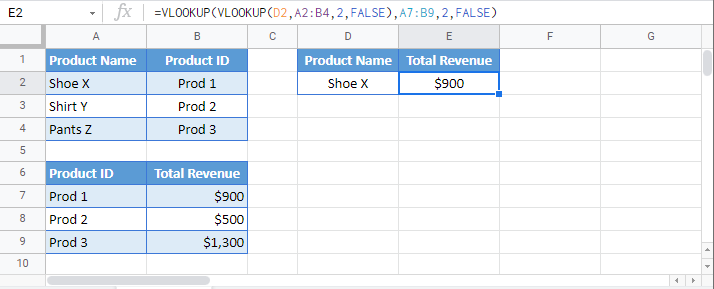### Excel Practice Worksheet

Practice Excel functions and formulas with our 100% free practice worksheets!